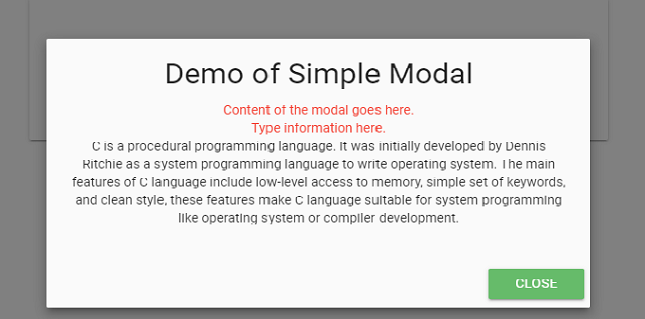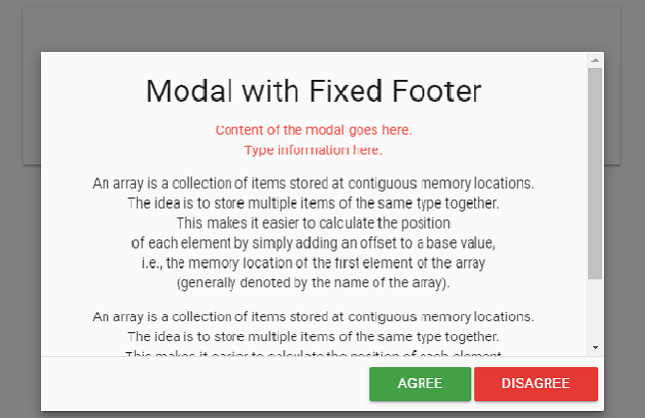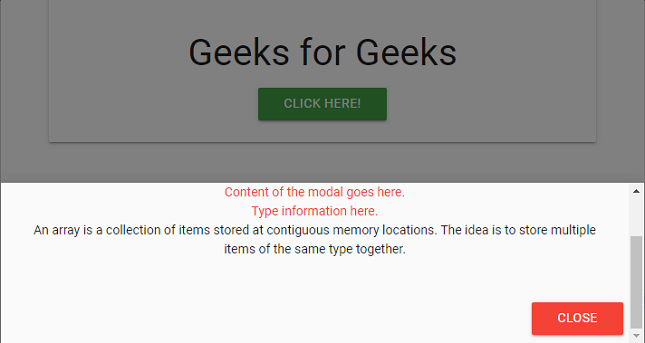GFG App
Open AppBrowser
Continue

Materialize CSS uses modal component for creating dialog boxes, confirmation messages or presenting important content to the viewers where underlying content becomes inactive until the modal window is closed.

In order for the modal to work, add the modal Id to the link of the trigger and include the reference materialize.min.js along with jQuery on the web page. To add a “close” button, add the class modal-close to your button.

Types of Modals:

1. Basic Modal: The basic modal consist of content and footer. The modal-content class is referred to the “main” div and the modal-footer class in another “div” tag where the “action” button is used.

Example:

## HTML

 ` ` `<``html``> ` ` `  `<``head``> ` `    ``<``link` `rel``=``"stylesheet"` `href``= ` `"https://cdnjs.cloudflare.com/ajax/libs/materialize/0.100.2/css/materialize.min.css"``> ` ` `  `    ``<``link` `href``= ` `"https://fonts.googleapis.com/icon?family=Material+Icons"` `rel``=``"stylesheet"``> ` `    ``<``script` `type``=``"text/javascript"` `src``= ` `        ``"https://code.jquery.com/jquery-2.1.1.min.js"``> ` `    `` ` `    ``<``script` `src``= ` `"https://cdnjs.cloudflare.com/ajax/libs/materialize/0.100.2/js/materialize.min.js"``> ` `    `` ` ` ` ` `  `<``body` `class``=``"container "``> ` `    ``<``div` `class``=``"card-panel center"``> ` `        ``<``h3``>Geeks for Geeks ` `        ``` `        ``<``a` `class="waves-effect waves-light btn  ` `            ``green darken-1 modal-trigger"  ` `            ``href``=``"#demo-modal"``> ` `            ``Click Here! ` `        `` ` ` `  `        ``` `        ``<``div` `id``=``"demo-modal"` `class``=``"modal"``> ` `            ``<``div` `class``=``"modal-content"``> ` `                ``<``h4``>Demo of Simple Modal ` `                ``<``p``> ` `                ``<``div` `class``=``"red-text"``> ` `                    ``Content of the modal goes here. <``br``> ` `                    ``Type information here. <``br``> ` `                `` ` `                ``C is a procedural programming language. ` `                ``It was initially developed by Dennis  ` `                ``Ritchie as a system programming language  ` `                ``to write operating system. The main  ` `                ``features of C language include low-level ` `                ``access to memory, simple set of keywords, ` `                ``and clean style, these features make C  ` `                ``language suitable for system programming  ` `                ``like operating system or compiler development. ` `                `` ` `            `` ` ` `  `            ``<``div` `class``=``"modal-footer"``> ` `                ``<``a` `href``=``"#!"` `class="modal-action  ` `                    ``modal-close waves-effect waves-green  ` `                    ``btn green lighten-1"> ` `                    ``Close ` `                `` ` `            `` ` `        `` ` `    `` ` `    ``<``script``> ` `        ``\$(document).ready(function () { ` `            ``\$('.modal').modal(); ` `        ``} ` `        ``) ` `    `` ` ` ` ` `  ` `

Output:2. Modal with Fixed Footer: If the content is large, then this type of modal is used. In this we can create “action” buttons that are fixed. For this, modal-fixed-footer class is used along with the modal class in the main container element.

Example:

## HTML

 ` ` `<``html``> ` ` `  `<``head``> ` `    ``<``link` `rel``=``"stylesheet"` `href``= ` `"https://cdnjs.cloudflare.com/ajax/libs/materialize/0.100.2/css/materialize.min.css"``> ` ` `  `    ``<``link` `href``= ` `"https://fonts.googleapis.com/icon?family=Material+Icons"` `rel``=``"stylesheet"``> ` `    ``<``script` `type``=``"text/javascript"` `src``= ` `        ``"https://code.jquery.com/jquery-2.1.1.min.js"``> ` `    `` ` `    ``<``script` `src``= ` `"https://cdnjs.cloudflare.com/ajax/libs/materialize/0.100.2/js/materialize.min.js"``> ` `    `` ` ` ` ` `  `<``body``> ` `    ``<``div` `class``=``"container center"``> ` `        ``<``div` `class``=``"card-panel"``> ` `            ``<``h3``>Geeks for Geeks ` `            ``` `            ``<``a` `class="waves-effect waves-light btn  ` `                ``green darken-1 modal-trigger"  ` `                ``href``=``"#demo-modal-fixed-footer"``> ` `                ``Click Here! ` `            `` ` ` `  `            ``` `            ``<``div` `id``=``"demo-modal-fixed-footer"`  `                ``class``=``"modal modal-fixed-footer"``> ` `                ``<``div` `class``=``"modal-content"``> ` `                    ``<``h4``>Modal with Fixed Footer ` `                    ``<``div` `class``=``"red-text"``> ` `                        ``Content of the modal goes here. <``br``> ` `                        ``Type information here. <``br``> ` `                    `` ` `                    ``<``p` `class``=``"center"``> ` `                        ``An array is a collection of items  ` `                        ``stored at contiguous memory locations.<``br``> ` `                        ``The idea is to store multiple items of  ` `                        ``the same type together.<``br``> This makes  ` `                        ``it easier to calculate the position<``br``> ` `                        ``of each element by simply adding an  ` `                        ``offset to a base value,<``br``> i.e., the  ` `                        ``memory location of the first element of ` `                        ``the array <``br``>(generally denoted by the  ` `                        ``name of the array).<``br``> ` `                    `` ` ` `  `                    ``<``p` `class``=``"center"``> ` `                        ``An array is a collection of items stored  ` `                        ``at contiguous memory locations.<``br``> ` `                        ``The idea is to store multiple items of  ` `                        ``the same type together.<``br``> ` `                        ``This makes it easier to calculate the  ` `                        ``position of each element <``br``> ` `                        ``by simply adding an offset to a base  ` `                        ``value, i.e., the memory location of <``br``> ` `                        ``the first element of the array (generally  ` `                        ``denoted by the name of the array).<``br``> ` `                    `` ` `                `` ` `                ``<``div` `class``=``"modal-footer"``> ` `                    ``<``a` `href``=``"#!"` `class="modal-action  ` `                        ``modal-close btn green darken-1"> ` `                        ``Agree ` `                    `` ` `                    ``<``a` `href``=``"#!"` `class="modal-action  ` `                        ``modal-close btn red darken-1"> ` `                        ``Disagree ` `                    `` ` `                `` ` `            `` ` `        `` ` `    `` ` `    ``<``script``> ` `        ``\$(document).ready(function () { ` `            ``\$('.modal').modal(); ` `        ``}) ` `    `` ` ` ` ` `  `                    `

Output:3. Bottom Sheet Modal: Modals can be displayed to the bottom of the user’s screen and not the middle. It is just like the normal modal that can be closed by clicking anywhere on the screen as well as using the button inside that modal. For this, bottom-sheet class is used along with the modal class in the <div> container.

Example:

## HTML

 ` ` `<``html``> ` ` `  `<``head``> ` `    ``<``link` `rel``=``"stylesheet"` `href``= ` `"https://cdnjs.cloudflare.com/ajax/libs/materialize/0.100.2/css/materialize.min.css"``> ` ` `  `    ``<``link` `href``= ` `"https://fonts.googleapis.com/icon?family=Material+Icons"` `rel``=``"stylesheet"``> ` `    ``<``script` `type``=``"text/javascript"`  `        ``src``=``"https://code.jquery.com/jquery-2.1.1.min.js"``> ` `    `` ` `    ``<``script` `src``= ` `"https://cdnjs.cloudflare.com/ajax/libs/materialize/0.100.2/js/materialize.min.js"``> ` `    `` ` ` ` ` `  `<``body` `class``=``"container "``> ` `    ``<``div` `class``=``"card-panel center"``> ` `        ``<``h3``>Geeks for Geeks ` `        ``` `        ``<``a` `class="waves-effect waves-light  ` `            ``btn green darken-1 modal-trigger"  ` `            ``href``=``"#demo-modal"``> ` `            ``Click Here! ` `        `` ` `        ``` `        ``<``div` `id``=``"demo-modal"` `class``=``"modal bottom-sheet"``> ` `            ``<``div` `class``=``"modal-content"``> ` `                ``<``h4``>Demo of Bottom sheet Modal ` `                ``<``p``> ` `                ``<``div` `class``=``"red-text"``> ` `                    ``Content of the modal goes here. <``br``> ` `                    ``Type information here. <``br``> ` `                `` ` `                ``An array is a collection of items stored at ` `                ``contiguous memory locations. ` `                ``The idea is to store multiple items of the ` `                ``same type together. ` `                `` ` `            `` ` `            ``<``div` `class``=``"modal-footer"``> ` `                ``<``a` `href``=``"#!"` `class=" modal-action  ` `                    ``modal-close waves-effect ` `                    ``waves-green btn red"> ` `                    ``Close ` `                `` ` `            `` ` `        `` ` `    `` ` `    ``<``script``> ` `        ``\$(document).ready(function () { ` `            ``\$('.modal').modal(); ` `        ``}) ` `    `` ` ` ` ` `  `         `

Output:My Personal Notes arrow_drop_up